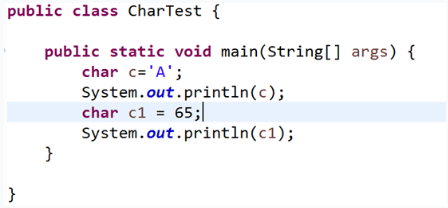# Java基础练习

## Java练习

Java基础练习

A. \$value B. Void C. class D. 1abc E. my value F.void_class

A. class B. package C. Void D. static

A. byte B. float C. boolean D. long

A. 数据类型分为基本数据类型和引用数据类型
B. 数组属于基本数据类型
C. 类属于引用数据类型
D. int和double属于基本数据类型

A. 使用“=”给变量进行赋值
B. 使用“==”给变量进行赋值
C. "="叫作赋值运算符，将运算符右边的值赋给左边的变量
D. “=“叫作赋值运算符，将运算符左边的值赋给右边的变量

A. 1.23 B. 1.23d C. 1.23D D.1.23f

A. double d=12.3d; B. double d=12.3D; C. float f=1.23f; D.float f=1.23;A. A B. 65 C. A 65 D.A A

A. ‘@’ B. ‘&’ C. “M” D.‘课’

A. “” B. ‘ab’ C. ‘a’ D.”\u0067”

A. char ch=65539; B. int n=5.6f; C. double d=1.2f; D. double d=1.23;float f=(float)d；

public class FloatDemo {
public static void main(String[] args) {
// 定义一个float类型的变量f1，值为98.4
float f1 = 98.4f;
//定义一个float类型的变量f2，将f1的值赋值给f2
float f2 = f1;
//定义一个整型变量n，值为55
int n = 55;
//定义一个double类型的变量d1，值为555.3
double d1 = 555.3;
//将n的值赋值给d1
d1 = n;
}
}


public class CharTest {

public static void main(String[] args) {
// 定义字符变量c，并赋值为'S'
char c = 'S';
//输出c的值
System.out.println(c);
//定义字符变量c1，并赋值为78
char c1 = 78;
//输出c1的值
System.out.println(c1);
}
}


public class StringDemo {
public static void main(String[] args) {
// 定义一个布尔类型的变量flag，赋值为true，并输出
boolean flag = true;
System.out.println(flag);
//定义一个字符串类型的变量str，值为"hello world"，并输出
String str = "hello world";
System.out.println(str);
//定义一个字符串类型的变量str1，值为空串
String str1 = "";
//将str的值赋值给str1，并输出
str1 = str;
System.out.println(str1);
}
}


public class TypeExchange {
public static void main(String[] args) {
// 定义一个整型变量n，值为98
int n = 98;
//定义一个char类型的变量ch，将变量n赋值给ch，然后输出ch的值
char ch = n;
System.out.println(ch);
//定义一个长整型变量var，值为190000000，并输出
long var = 190000000L;
System.out.println(var);
//定义一个double类型的变量d，将变量var的值赋值给d
double d = var;
}
}


public class TypeExchange {
public static void main(String[] args) {
// 将一个整型字面值67832赋值给char类型变量c
//并将c的值输出
char c = (char)67832;
System.out.println(c);
//定义一个整型变量n，值为65
int n = 65;
//定义一个字符型变量c1，赋值为n，并输出c1的值
char c1 = (char)n;
System.out.println(c1);
//定义一个长整型变量l，值为987654321
long l = 987654321L;
//定义一个整型变量i，赋值为l，并输出i的值
int i = (int)l;
System.out.println(i);
//定义一个float类型变量f，将变量l的值赋值给f，并输出f的值
float f = l;
System.out.println(f);
//将float的值f，重新赋值给变量l，并输出l的值
long l =(long) f;
System.out.println(l);
}
}04-16
08-202285
12-08705
08-0122万+
08-12843
12-021250
03-25398
12-021077
12-031648
03-279404
12-241538
06-059911
©️2020 CSDN 皮肤主题: 1024 设计师:白松林